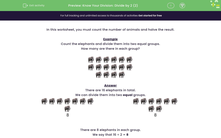# Divide Groups of Animals By 2

In this worksheet, students will count the number of animals and halve the result. This means that they divide by 2.Key stage:  KS 1

Curriculum topic:   Number: Multiplication and Division

Curriculum subtopic:   Use Multiplication/Division Facts (2, 5, 10)

Popular topics:   Multiplication worksheets, Division worksheets

Difficulty level:#### Worksheet Overview

In this activity, we will be counting the number of animals and halving the result.

Example

Count the elephants and divide them into two equal groups.

How many are there in each group?There are 16 elephants in total.

We can divide them into two equal groups.8 8

There are 8 elephants in each group.

So half of 16 is 8.

We say that 16 ÷ 2 = 8

Does that make sense? If so, let's move on to the questions.### What is EdPlace?

We're your National Curriculum aligned online education content provider helping each child succeed in English, maths and science from year 1 to GCSE. With an EdPlace account you’ll be able to track and measure progress, helping each child achieve their best. We build confidence and attainment by personalising each child’s learning at a level that suits them.

Get started